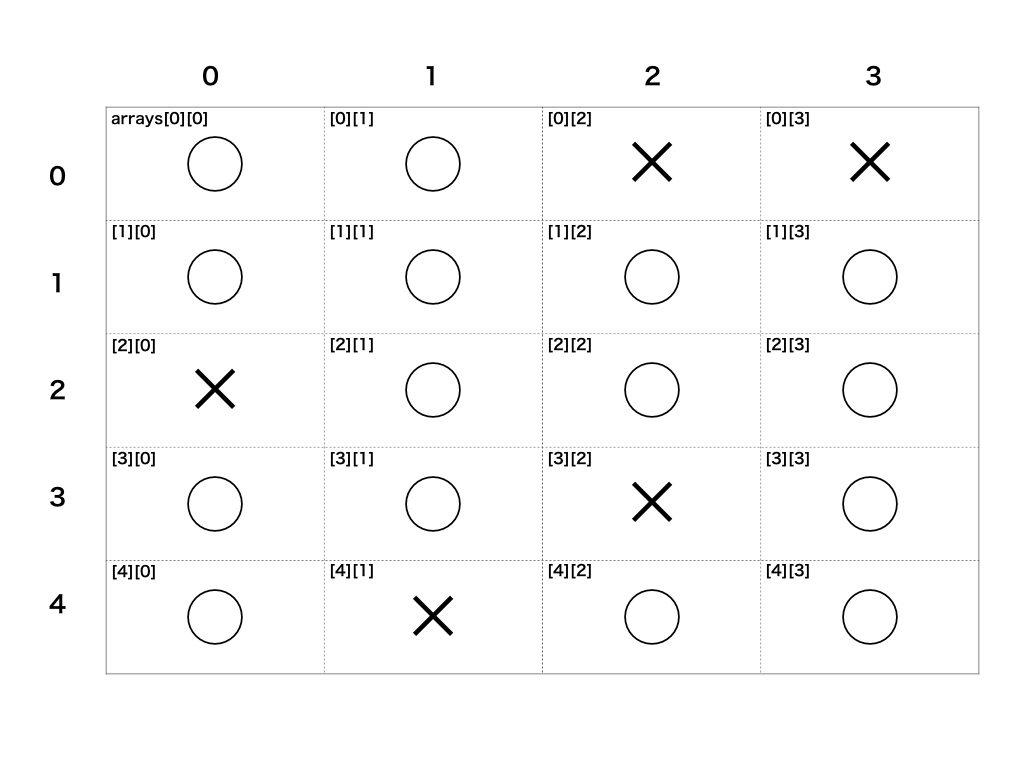# What is a two-dimensional array?

A two-dimensional array is an array that has an array as an element.

``````#Example:
number_arrays = [[1, 2, 3], [4, 5, 6], [7, 8, 9]]
color_arrays = [["red", "yellow", "blue"], ["white", "black", "gray"]]
``````

Two-dimensional (planar) data can be represented by using a two-dimensional array. Example: Expressing the state of squares (◯ or ✕) in a two-dimensional array``````# ◯：1,✕: Set to 0
arrays = [
[1, 1, 0, 0],
[1, 1, 1, 1],
[0, 1, 1, 1],
[1, 1, 0, 1],
[1, 0, 1, 1]
]
``````

# How to use a two-dimensional array

## Creating a two-dimensional array

A two-dimensional array can be created in the same way as a normal array.

``````#Directly define and create
arrays = [[1, 2, 3], [4, 5, 6], [7, 8, 9]]

#Define an empty array arrays and add an array in it to create
arrays = []
arrays << [1, 2, 3]
arrays << [4, 5, 6]
arrays << [7, 8, 9]

arrays #=> [[1, 2, 3], [4, 5, 6], [7, 8, 9]]
``````

## Get value

To get an array in a two-dimensional array ** Variable name [index number of array to get] ** Describe as.

``````arrays = [[1, 2, 3], [4, 5, 6], [7, 8, 9]]

arrays #=> [1, 2, 3]
arrays #=> [4, 5, 6]
arrays #=> [7, 8, 9]
``````

Also, to get each element of an array in a two-dimensional array ** Variable name [Index number of array to be acquired] [Index number of element to be acquired] ** Describe as.

``````arrays = [[1, 2, 3], [4, 5, 6], [7, 8, 9]]

arrays #=> 1
arrays #=> 2
arrays #=> 3
arrays #=> 4
arrays #=> 5
arrays #=> 6
arrays #=> 7
arrays #=> 8
arrays #=> 9
``````

# Methods for 2D arrays

There are also methods for two-dimensional arrays. transpose You can use the transpose method to create a 2D array with the rows and columns of the 2D array swapped.

``````#Example:

arrays = [[1, 2, 3], [4, 5, 6], [7, 8, 9]]

#The array is represented by a plane as follows.
# arrays = [
#   [1, 2, 3],
#   [4, 5, 6],
#   [7, 8, 9]
# ]

transposed_arrays = arrays.transpose
transposed_arrays #=> [[1, 4, 7], [2, 5, 8], [3, 6, 9]]

# transposed_The array is represented by a plane as follows.
# transposed_arrays = [
#   [1, 4, 7],
#   [2, 5, 8],
#   [3, 6, 9]
# ]
``````

flatten You can use the flatten method to create an array that flattens a two-dimensional array (separates the array inside).

``````#Example:

arrays = [[1, 2, 3], [4, 5, 6], [7, 8, 9]]
flattened_arrays = arrays.flatten
flattened_arrays #=> [1, 2, 3, 4, 5, 6, 7, 8, 9]
``````

# Summary

• A two-dimensional array is an array that has an array as an element.
• Planar data can be represented using a two-dimensional array
• Arrays and values in 2D arrays can be obtained by specifying the index number.
• Some methods can be used for 2D arrays such as transpose and flatten